# RS Aggarwal Solutions for Class 9 Chapter 2: Polynomials Exercise 2C

## RS Aggarwal Solutions for Class 9 Maths Exercise 2C PDF

Regular practice on a daily basis is the main thing that should be done by the students in Mathematics field. Highly experienced subject experts having a vast knowledge of concepts prepare the answers which match the understanding ability of the students. RS Aggarwal Solutions for Class 9 can be used by students in order to excel in the subject by obtaining a good academic score. This exercise covers the topic division algorithm in polynomials with explanatory answers. RS Aggarwal Solutions for Class 9 Maths Chapter 2 Polynomials Exercise 2C are provided here.

## RS Aggarwal Solutions for Class 9 Chapter 2: Polynomials Exercise 2C Download PDF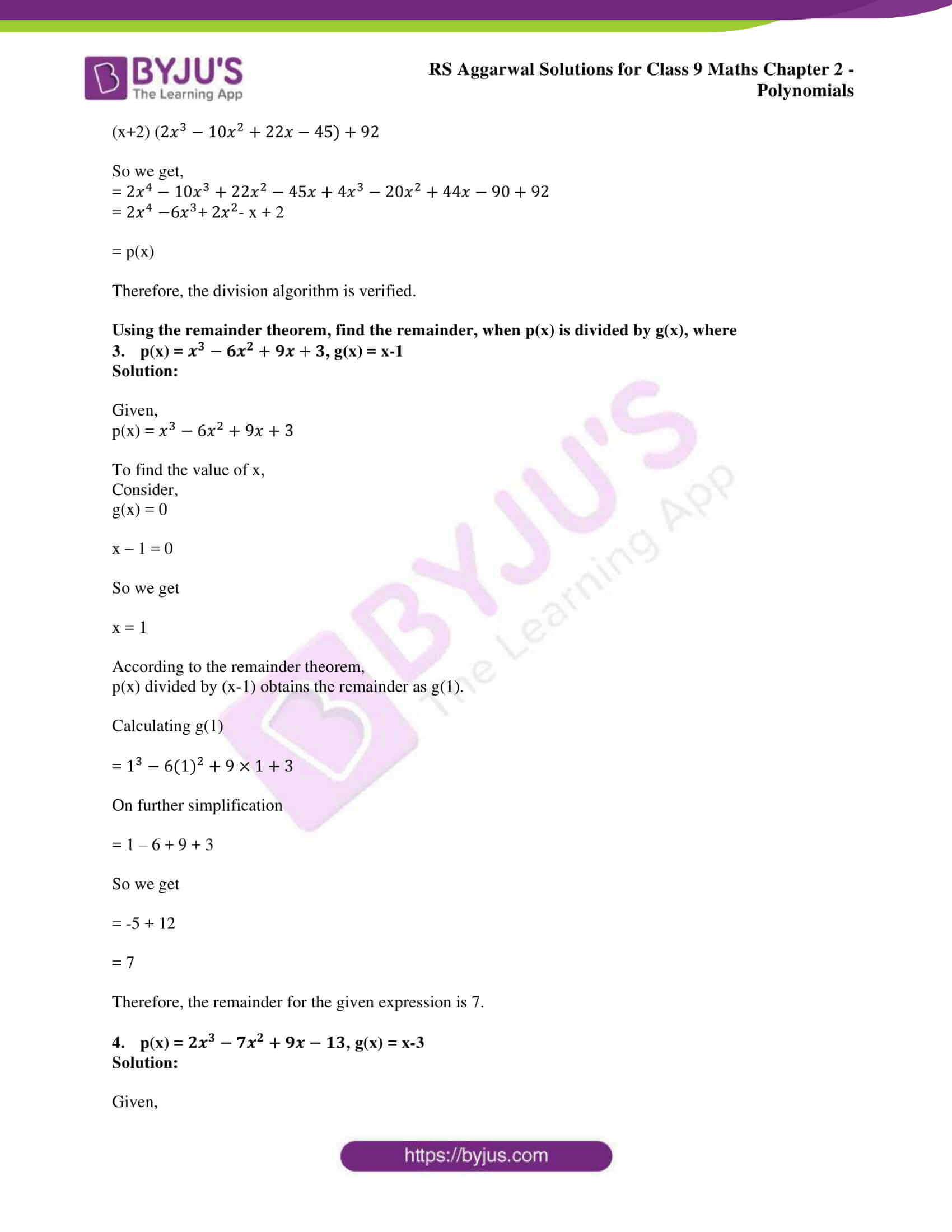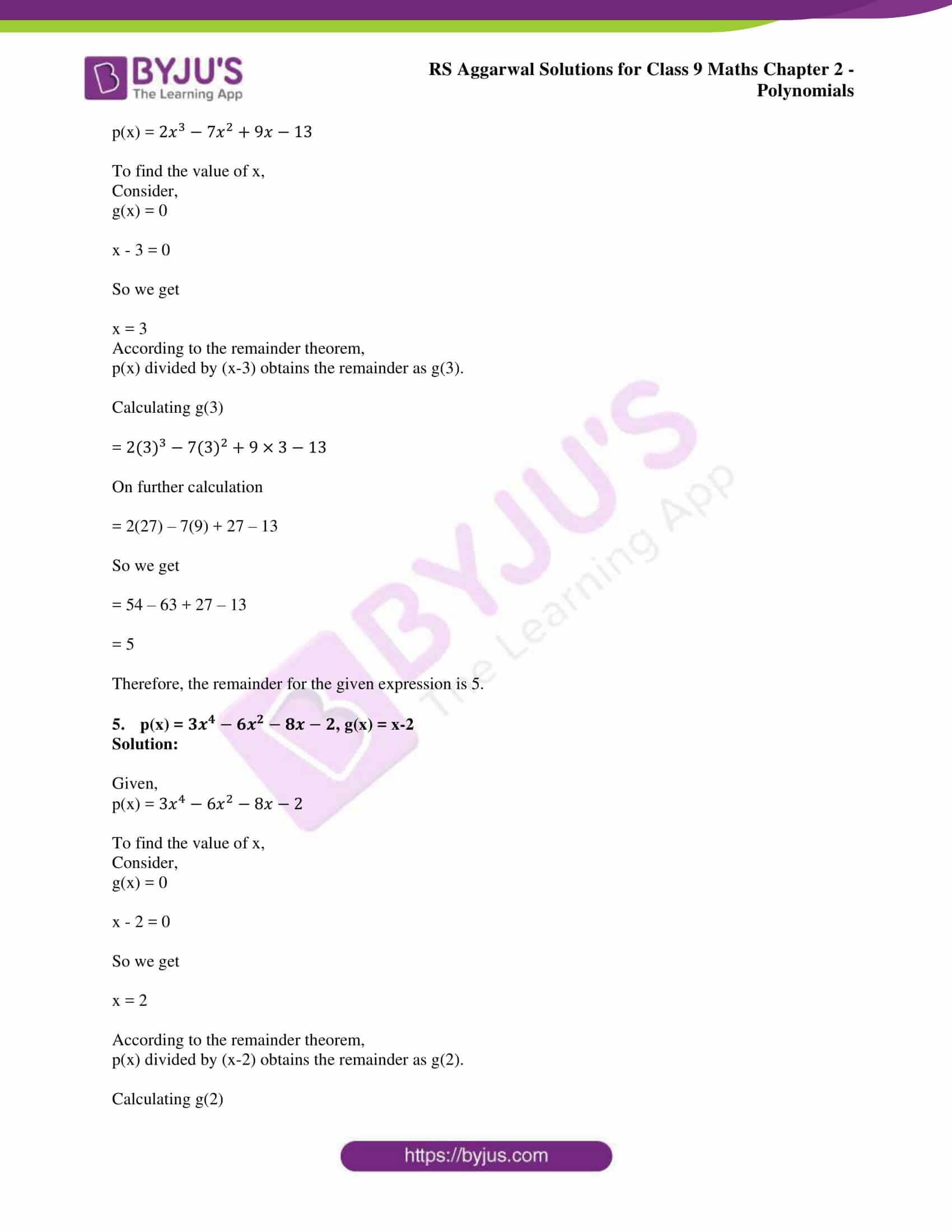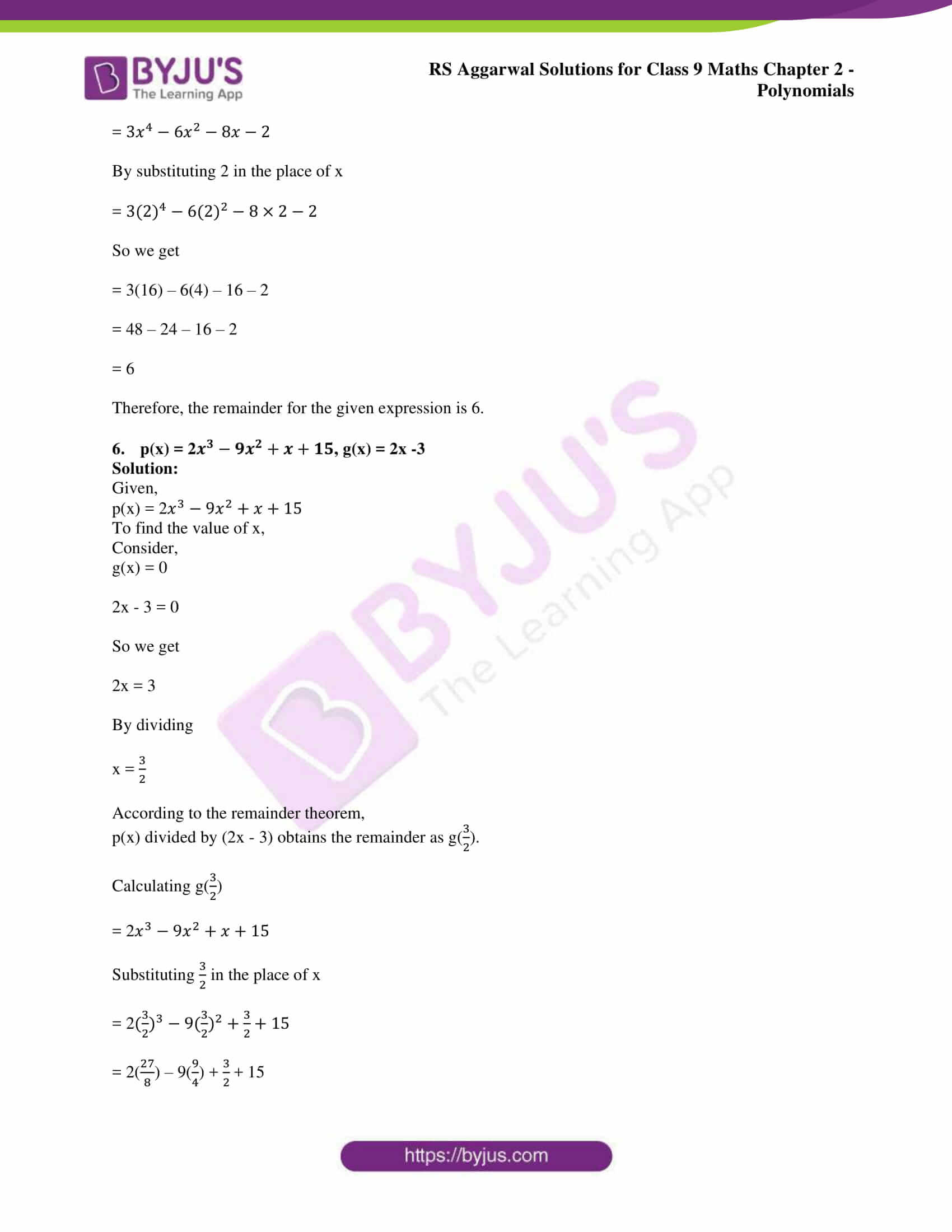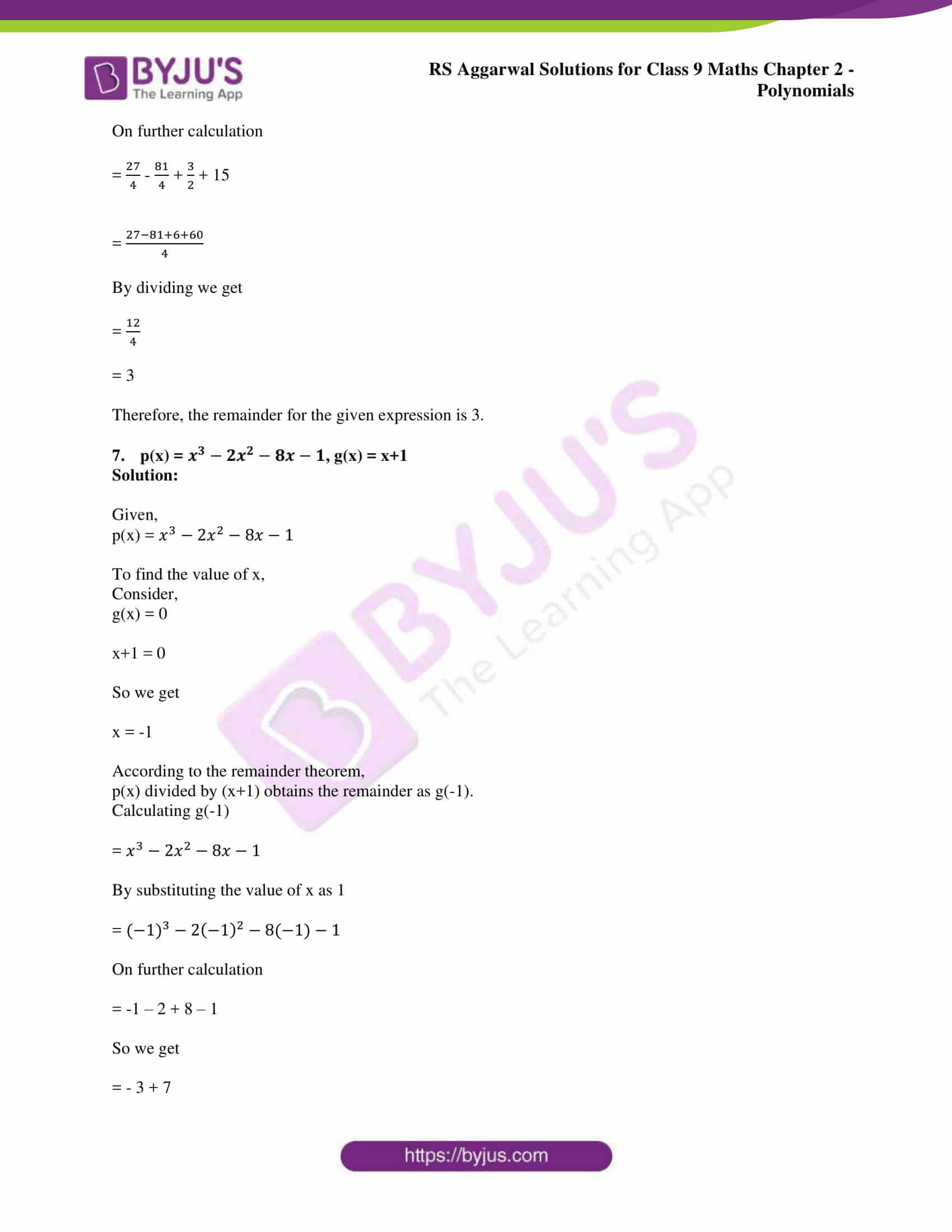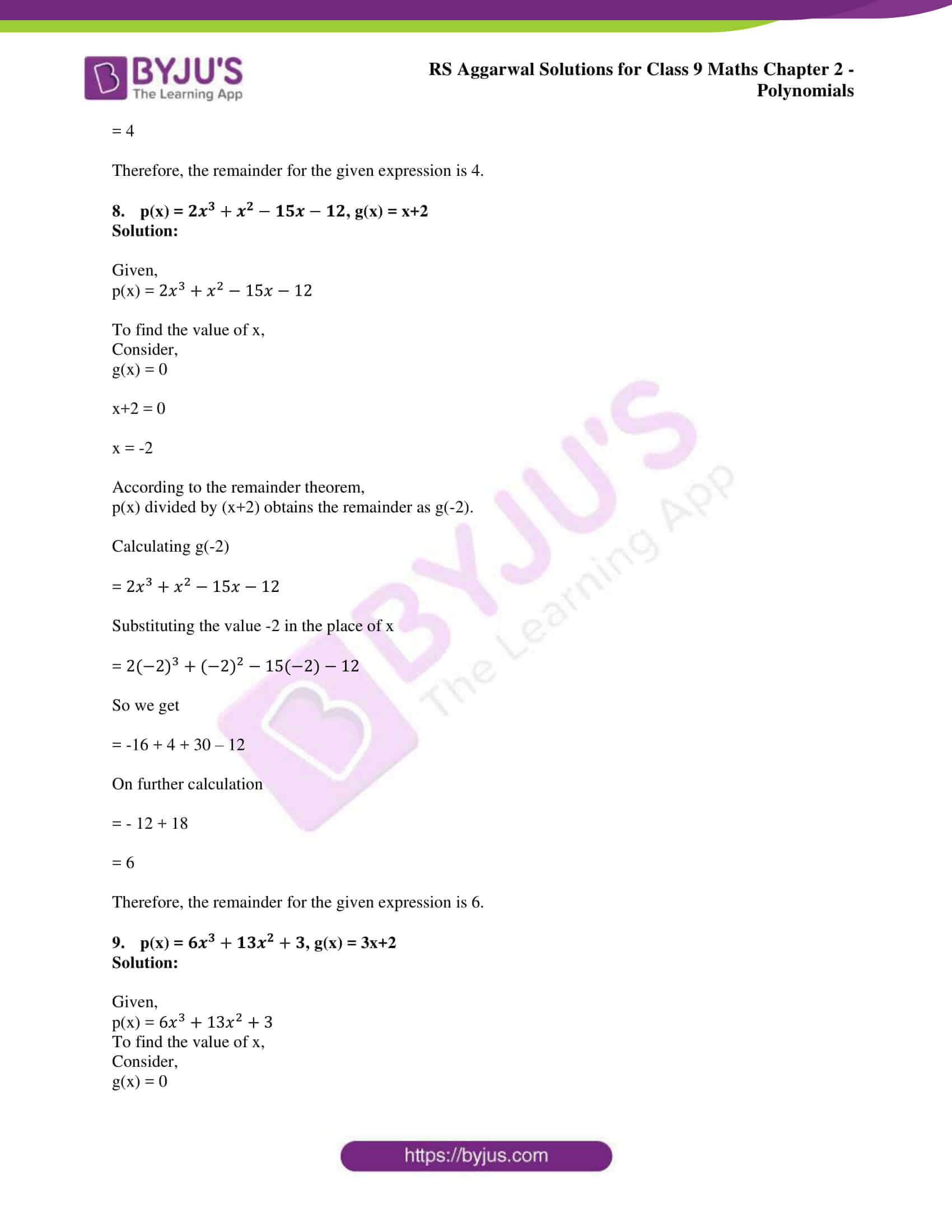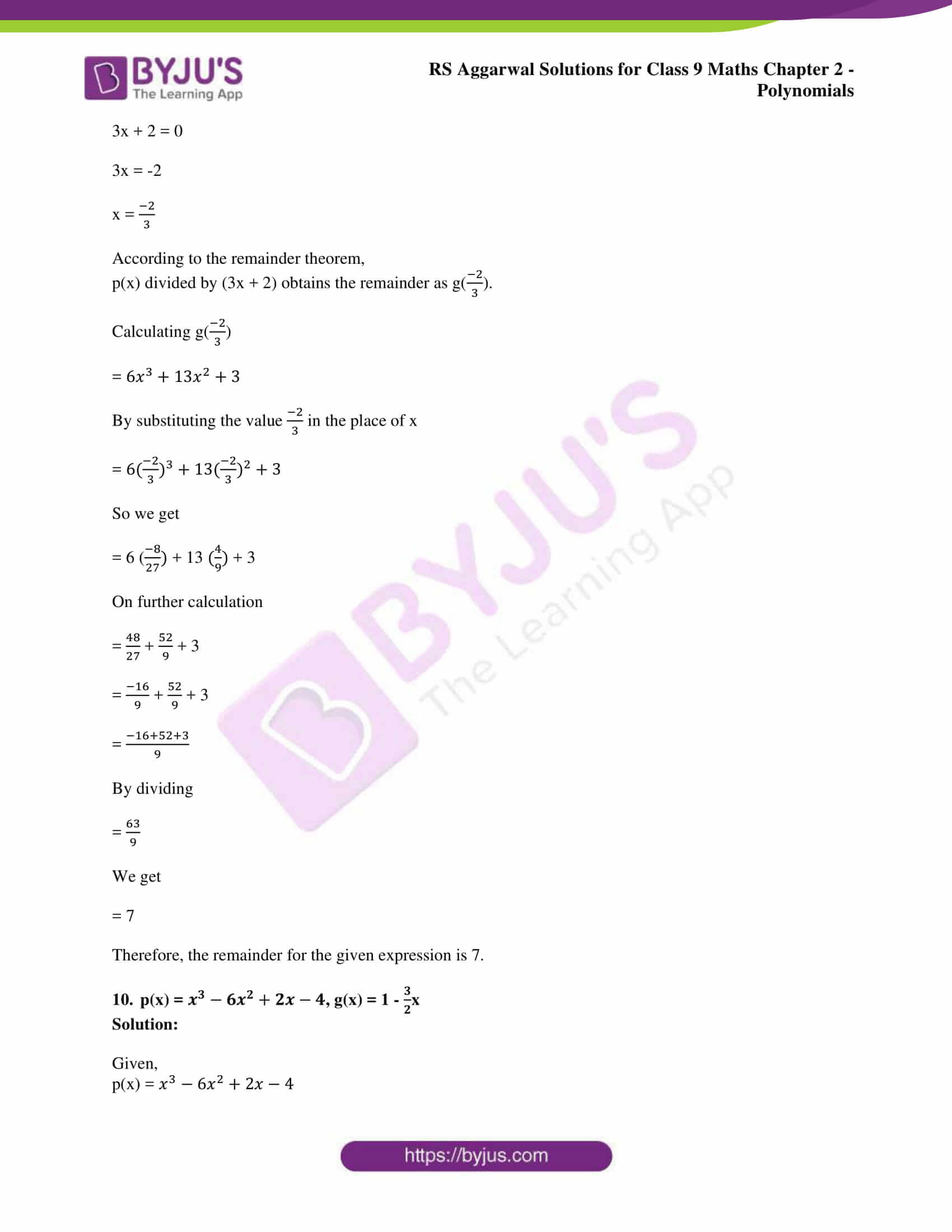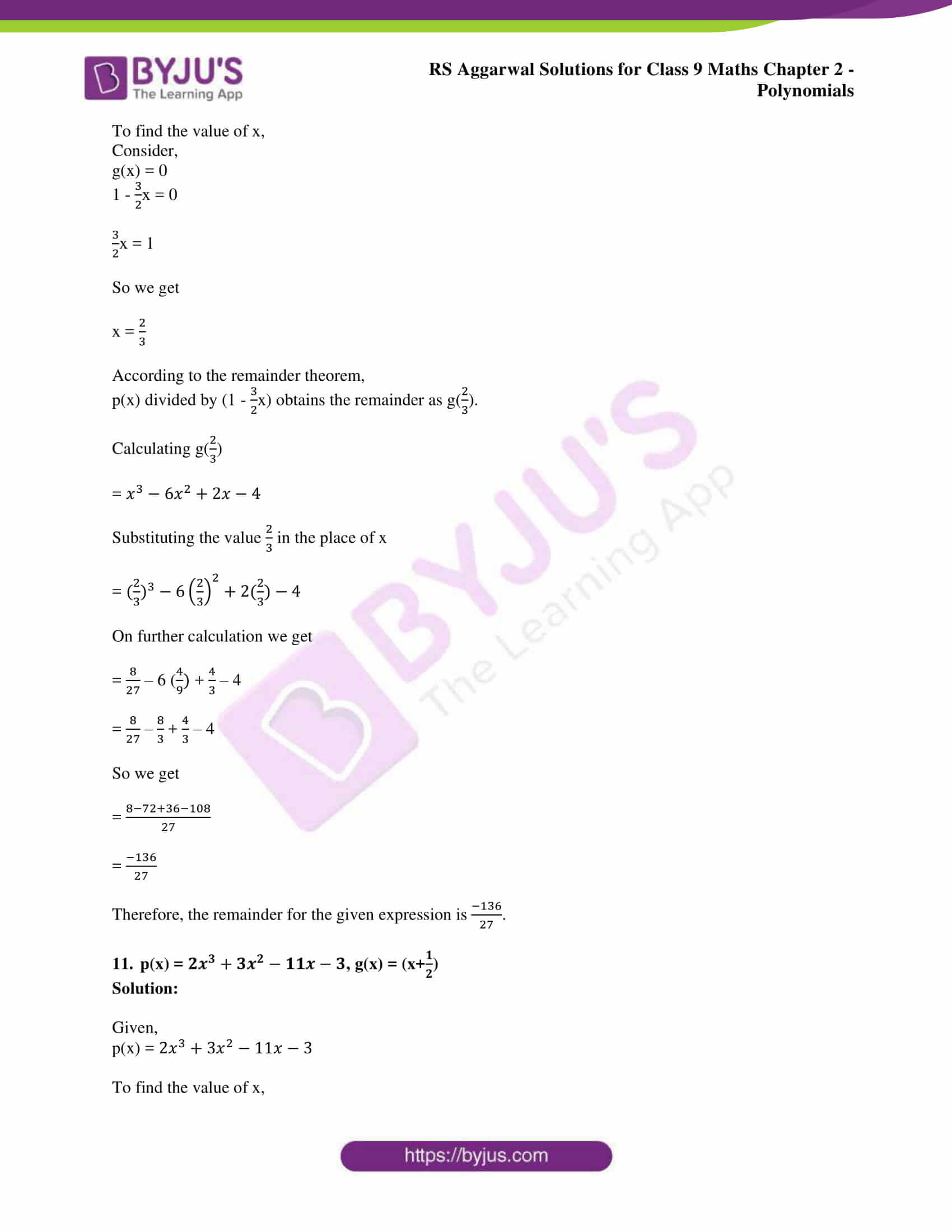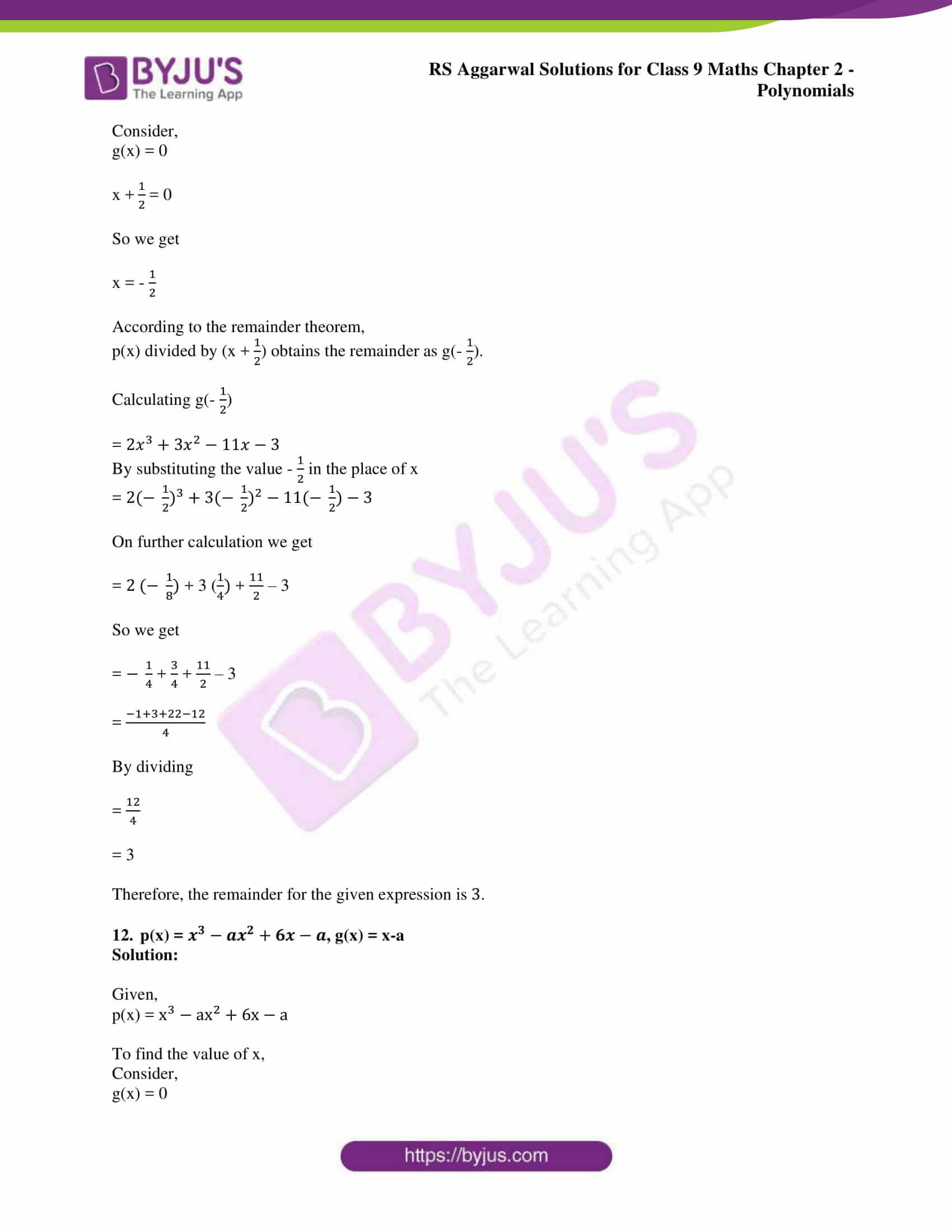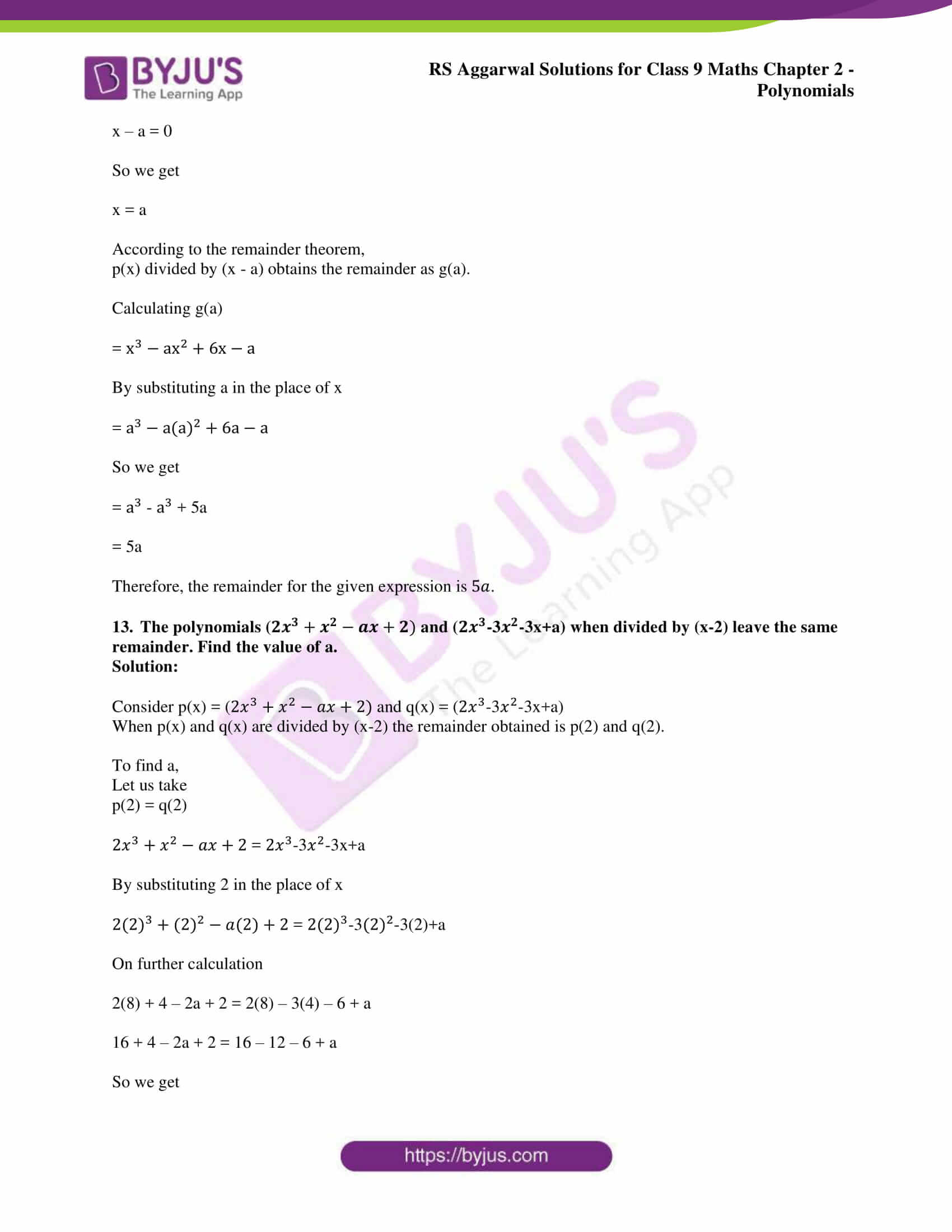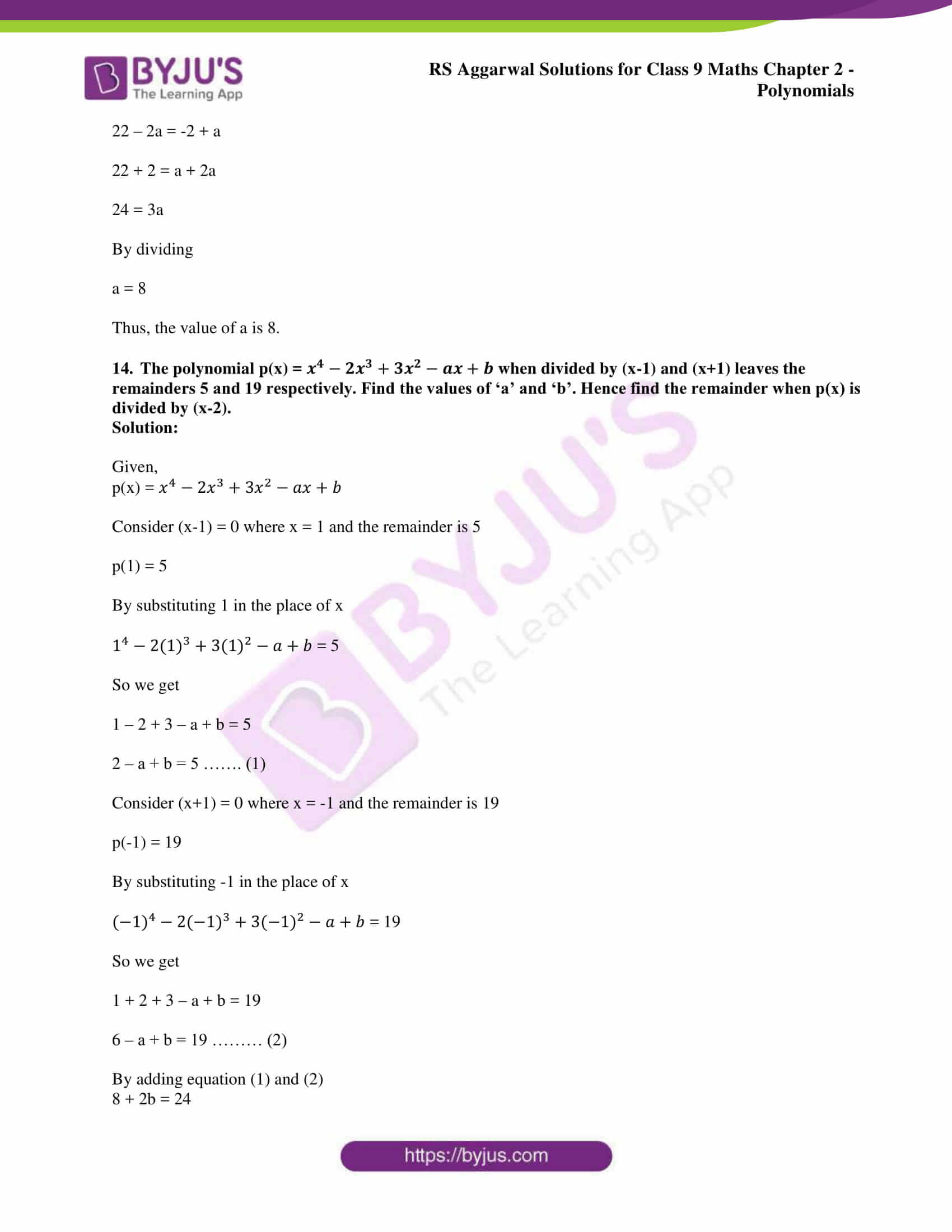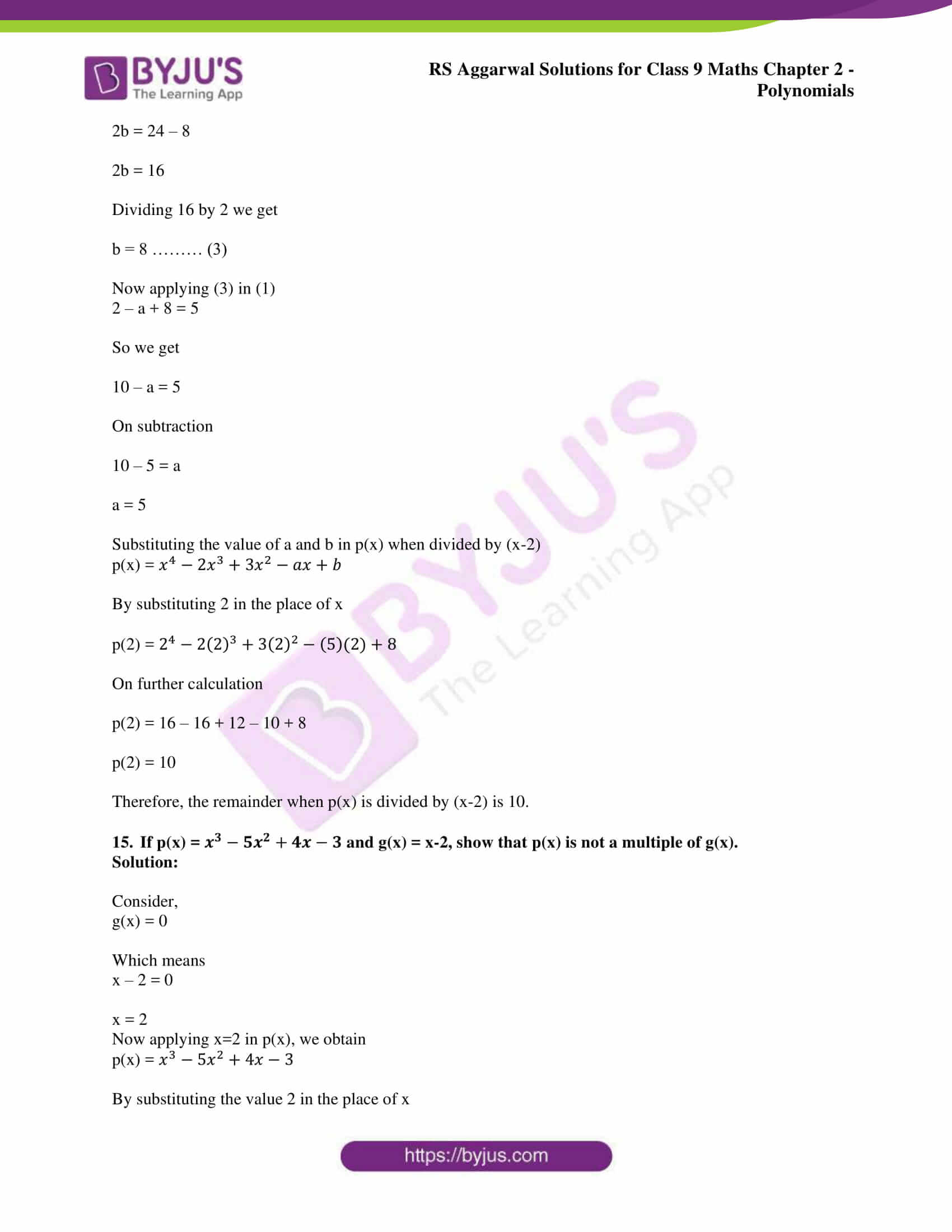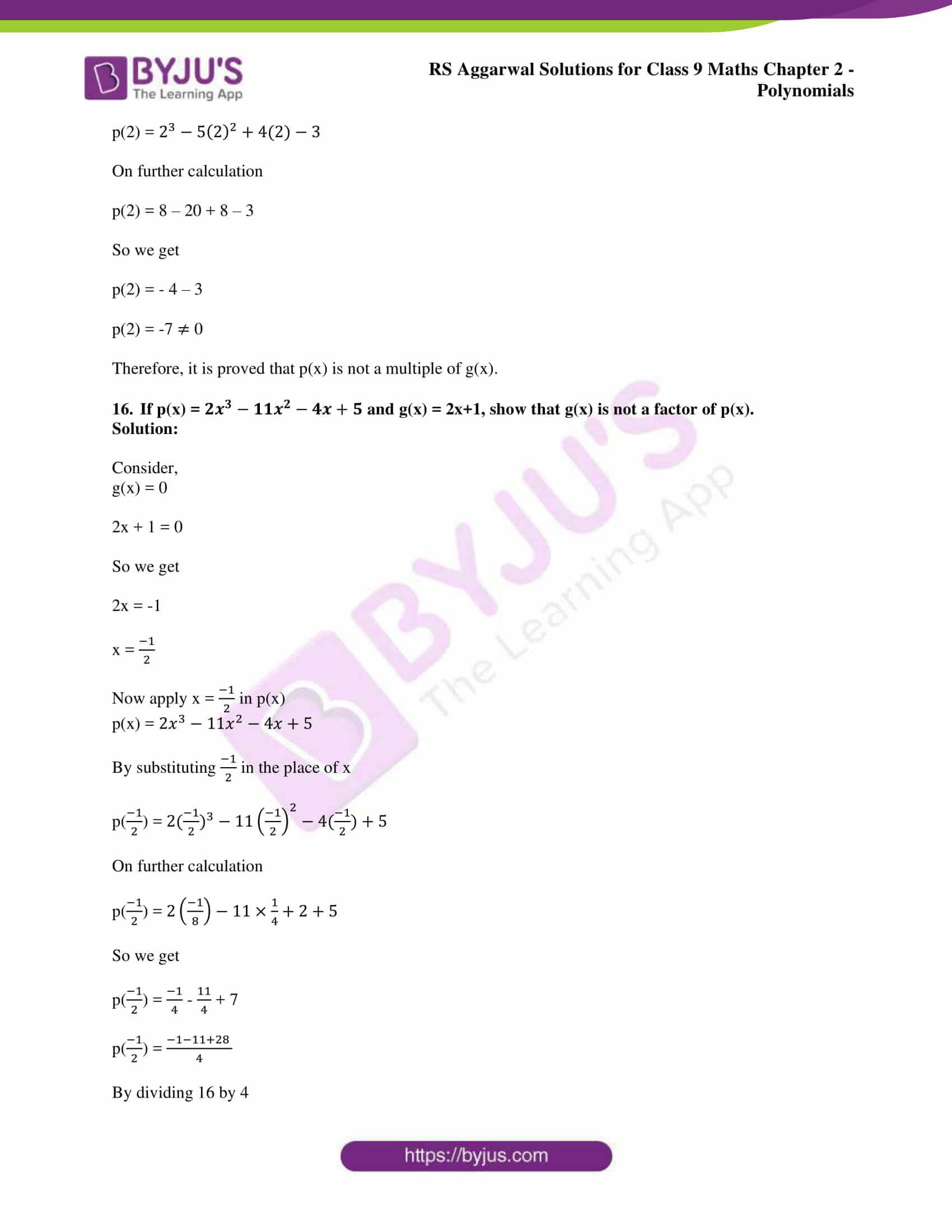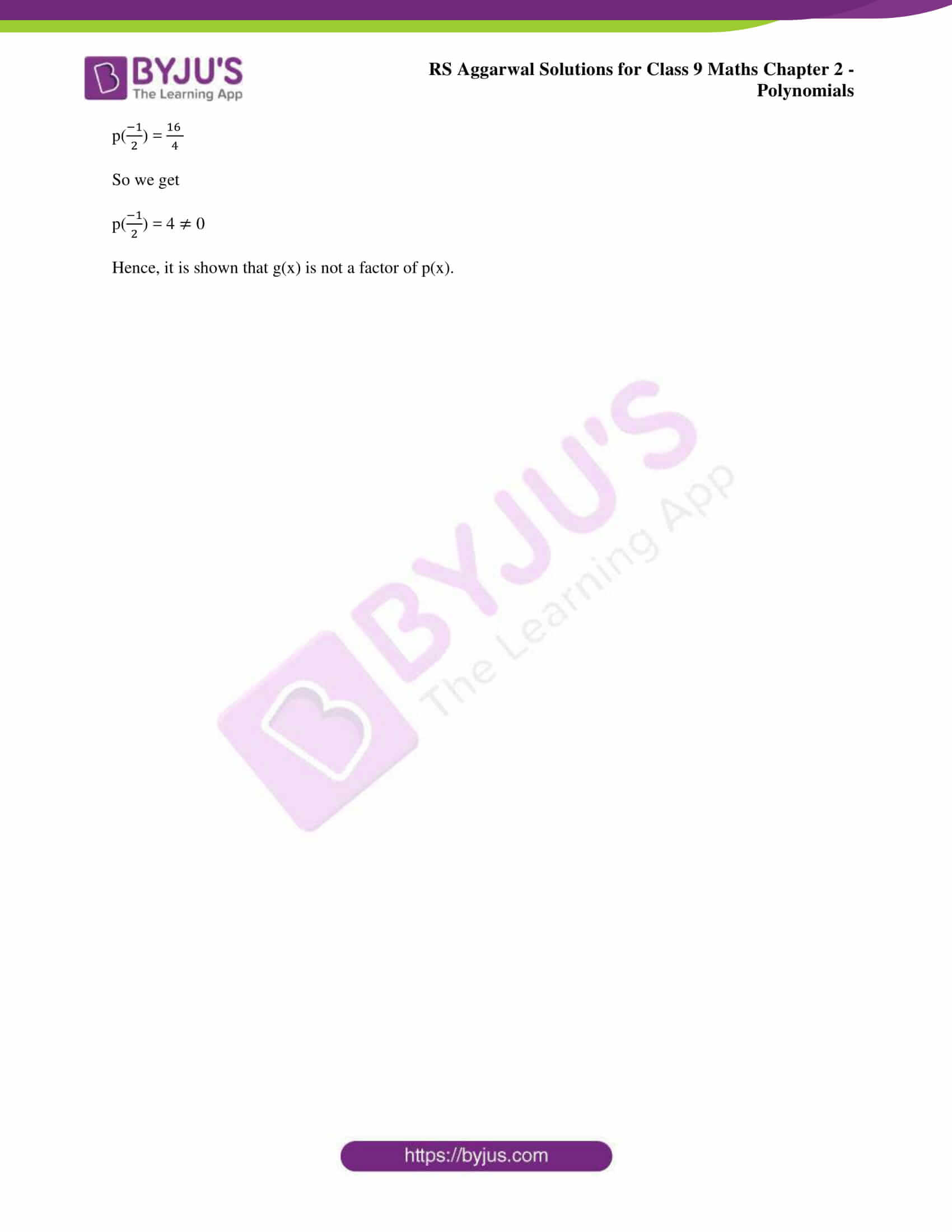### Access other exercise solutions of class 9 Maths Chapter 2: Polynomials

Exercise 2A Solutions 6 Questions

Exercise 2B Solutions 9 Questions

Exercise 2D Solutions 26 Questions

### RS Aggarwal Solutions Class 9 Maths Chapter 2 – Polynomials Exercise 2C

RS Aggarwal Solutions Class 9 Maths Chapter 2 Polynomials Exercise 2C explains in brief about the various methods used in solving problems based on the Division Algorithm in Polynomials.

### Key features of RS Aggarwal Solutions for Class 9 Maths Chapter 2: Polynomials Exercise 2C

• RS Aggarwal Solutions can be used by the students as a reference material for revision purpose for exam preparation.
• Solving the problems based on RS Aggarwal textbook can increase the confidence level in students to appear for the exams.
• The solutions are prepared in such a way that even a student who is weak in Mathematics can manage to clear the exam.
• Many number of methods are used to solve the problems so that it makes it simple for students to solve difficult problems easily.